### Group Theory

So far, we’ve been looking at symmetries of two-dimensional shapes. In this quiz, we’ll investigate the rotational symmetries of a three-dimensional object: the cube! (If you have a cube lying around, you should get it before continuing! A regular die is perfect.)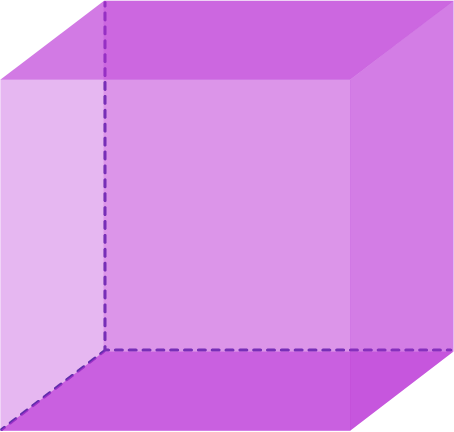Remember that we’re looking at the symmetries of an object, which are the rigid transformations that send the object to itself (that is, essentially leave it unchanged, or in the case of the identity transformation, completely leave it unchanged). In this quiz we’re going to investigate all the ways you can rotate a cube into itself. For example, you can rotate a cube by $90^{\circ}$ about an axis that goes through the centers of the top and the bottom sides.

To begin, how many different rotations do you think there are of the cube? Remember that we’re only interested in rotations that send the cube back to itself. So, using the axis that goes through the top and bottom sides, we’re interested in rotations by $90^{\circ},$ $180^{\circ}$ and $270^{\circ},$ but not by $45^{\circ}.$ Of course, there are other possible axes...

The number of different rotational symmetries of the cube is:

# Cube Symmetries

It turns out that there are 24 rotational symmetries of the cube, and we’re going to find them all! (This number includes the identity, which we will think of as a rotation by $0^{\circ}.$ Our strategy will be to count the 23 non-identity rotations, and then include the identity.)

We’ll start with the easiest kind to visualize: those with a rotational axis that joins the centers of two opposite sides. We will call these Type I rotations.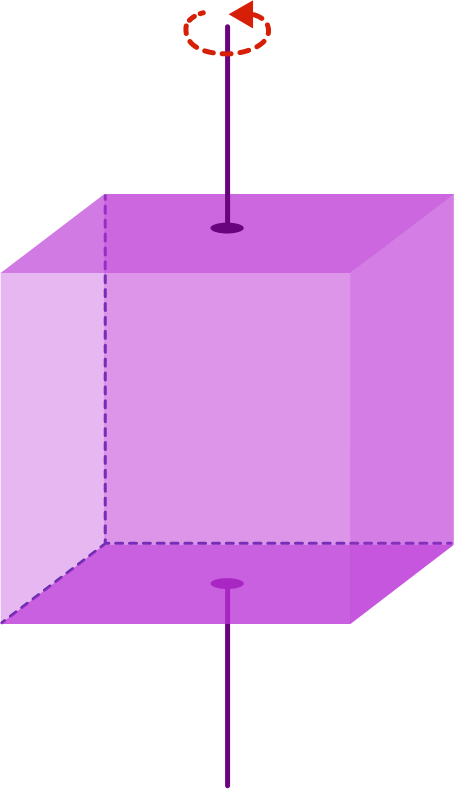We can rotate about this axis by $90^{\circ},$ $180^{\circ},$ or $270^{\circ},$ so this axis gives us three rotations. (Of course, we can also rotate by $360^{\circ},$ but that’s the same as the identity, which we're not counting yet.)

So we have 3 rotations, but those aren’t all the Type I rotations, because there are other axes that join the centers of opposite sides, like this one:How many Type I rotations are there in total? (Don’t count the identity rotation.)

# Cube Symmetries

So far we have 9 rotations! The next kind are a little harder to visualize.

A Type II rotation is a rotation about an axis that joins two opposite corners of the cube: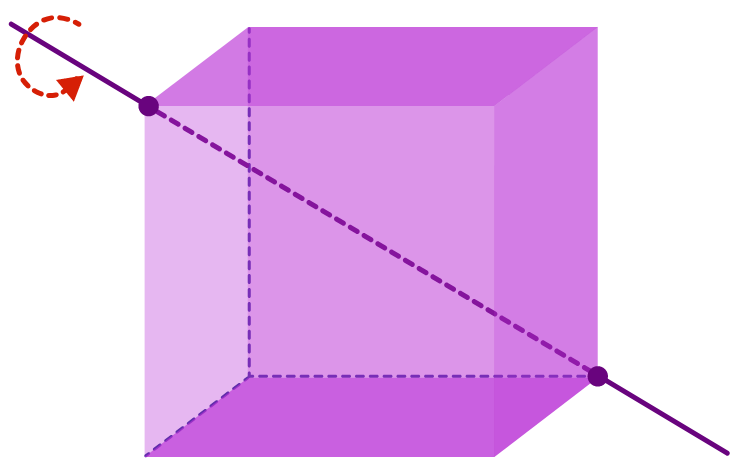For Type I rotations, we saw that the allowable angles of rotation were $90^{\circ},$ $180^{\circ}$ and $270^{\circ}.$ What are the allowable angles for Type II? Here's a helpful visualization trick: Visualize the rotation with the axis pointing directly at you. This gives a picture like this: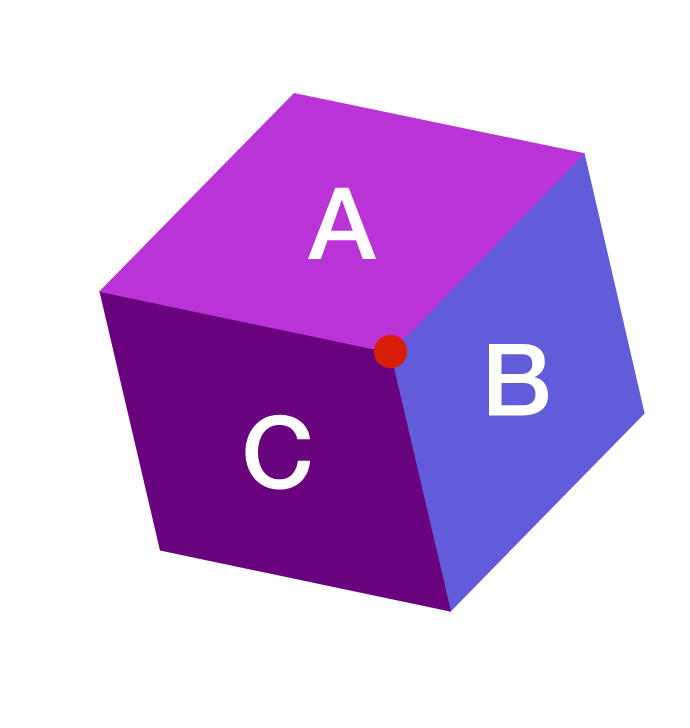From this perspective, it should be clear that when we do a Type II rotation, the three faces we see in the picture all change places; for instance, A might move to B, B to C, and C back to A.

What are the rotational angles used with Type II rotations?

# Cube Symmetries

So for each Type II axis, we have two rotations, by $120^{\circ}$ and $240^{\circ}.$ But there is more than one possible axis. What is the total number of Type II rotations? (Again, don’t include the identity rotation.)

# Cube Symmetries

So far, we have 9 Type I rotations and 8 Type II rotations, which is 17. We're looking for 23 non-identity rotations, so we're missing 6.

As a challenge, before going on, pick up your cube and try figuring out what axes we’re missing. How are we going to define Type III rotations on the next page?

# Cube Symmetries

Did you find the missing rotations? Here’s one way to think about it: the Type I rotation axes connected the centers of opposite faces, and the Type II rotations connected opposite vertices. Perhaps we should consider the possibility of axes that connect opposite edges.

So, a Type III rotation of the cube will be a rotation about the axis that joins the centers of two opposite edges, as in this picture: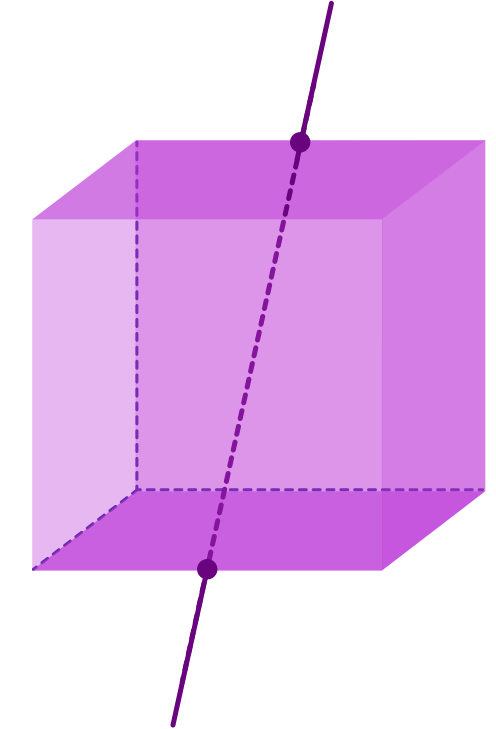These are perhaps the hardest to visualize, so let’s again look at them with the axis pointing at us, which means that we’re looking directly at an edge: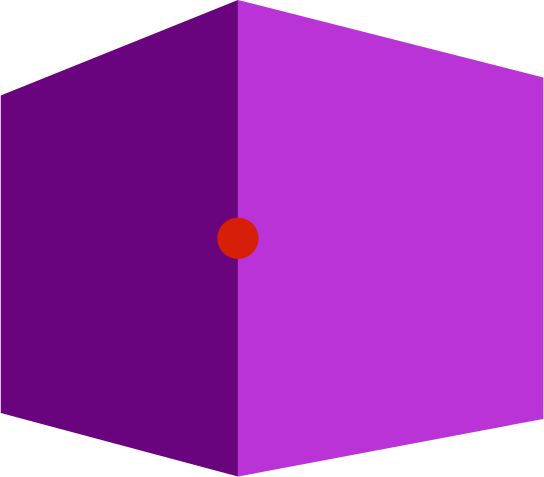From this picture, we see that the rotation must swap the two faces adjoining the edge in the center. So the only allowable rotation is by $180^{\circ}.$ As before, though, there is more than one Type III axis. How many (non-identity) Type III rotations are there? (Hint: How many edges are there?)

# Cube Symmetries

We’ve found the missing six rotations, which means that we’ve found all 24: 9 of Type I, 8 of Type II, 6 of Type III, and the identity. A couple of closing points in the form of questions and answers.

We’ve found 24 rotations. How do we know there aren’t more?

Here’s a sketch of an argument. A cube has four diagonals, the line segments joining a vertex with the opposite vertex. Here’s a picture of them.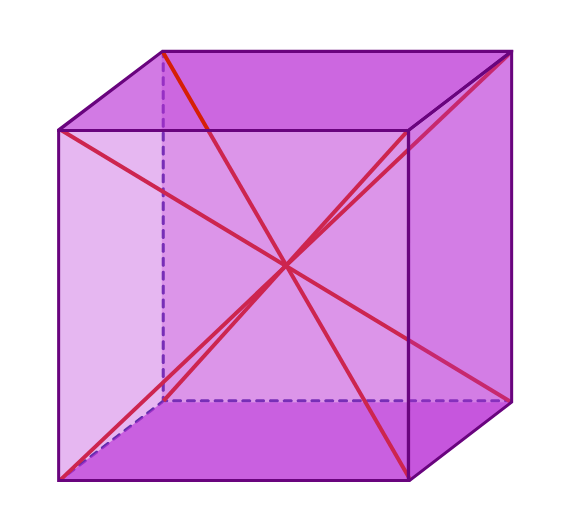The critical point is that every rotation of the cube permutes the four diagonals, and, what’s more, different rotations yield different permutations of the diagonals. This isn’t obvious, especially that second part, but you might think about and see why it’s true. (This is why this is just a “sketch” of an argument!)

Now, there are 24 permutations of 4 objects. Since each rotation of the cube gives a different permutation of the diagonals, there can’t be more than 24 rotations of the cube. That’s how we know there aren’t more.

# Cube Symmetries

What about other symmetries… what about reflections?

Yes, there are other symmetries of the cube; i.e. other rigid transformations that send the cube to itself. For instance, you can reflect about a plane that bisects the cube. It turns out there are exactly 24 non-rotation symmetries, and they’re all reflections.

What about the “multiplication” on all these symmetries? That sounds like it’s going to be really complicated.

Well, one of the cool things about group theory is that we’ll have a framework for understanding these complicated objects. Eventually we’ll see that the multiplication table for rotations corresponds to a group we’ll call $S_4,$ which will become an old friend. Keep going to make its acquaintance!

×